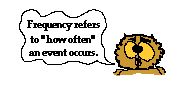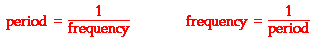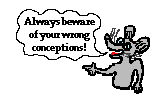Vibrations and Waves - Lesson 2 - Properties of a Wave

# Frequency and Period of a Wave

The nature of a wave was discussed in Lesson 1 of this unit. In that lesson, it was mentioned that a wave is created in a slinky by the periodic and repeating vibration of the first coil of the slinky. This vibration creates a disturbance that moves through the slinky and transports energy from the first coil to the last coil. A single back-and-forth vibration of the first coil of a slinky introduces a pulse into the slinky. But the act of continually vibrating the first coil with a back-and-forth motion in periodic fashion introduces a wave into the slinky.

Suppose that a hand holding the first coil of a slinky is moved back-and-forth two complete cycles in one second. The rate of the hand's motion would be 2 cycles/second. The first coil, being attached to the hand, in turn would vibrate at a rate of 2 cycles/second. The second coil, being attached to the first coil, would vibrateat a rate of 2 cycles/second. The third coil, being attached to the second coil, would vibrate at a rate of 2 cycles/second. In fact, every coil of the slinky would vibrate at this rate of 2 cycles/second. This rate of 2 cycles/second is referred to as the frequency of the wave. The frequency of a wave refers to how often the particles of the medium vibrate when a wave passes through the medium. Frequency is a part of our common, everyday language. For example, it is not uncommon to hear a question like "How frequently do you mow the lawn during the summer months?" Of course the question is an inquiry about how often the lawn is mowed and the answer is usually given in the form of "1 time per week." In mathematical terms, the frequency is the number of complete vibrational cycles of a medium per a given amount of time. Given this definition, it is reasonable that the quantity frequency would have units of cycles/second, waves/second, vibrations/second, or something/second. Another unit for frequency is the Hertz (abbreviated Hz) where 1 Hz is equivalent to 1 cycle/second. If a coil of slinky makes 2 vibrational cycles in one second, then the frequency is 2 Hz. If a coil of slinky makes 3 vibrational cycles in one second, then the frequency is 3 Hz. And if a coil makes 8 vibrational cycles in 4 seconds, then the frequency is 2 Hz (8 cycles/4 s = 2 cycles/s).

The quantity frequency is often confused with the quantity period. Period refers to the time that it takes to do something. When an event occurs repeatedly, then we say that the event is periodic and refer to the time for the event to repeat itself as the period. The period of a wave is the time for a particle on a medium to make one complete vibrational cycle. Period, being a time, is measured in units of time such as seconds, hours, days or years. The period of orbit for the Earth around the Sun is approximately 365 days; it takes 365 days for the Earth to complete a cycle. The period of a typical class at a high school might be 55 minutes; every 55 minutes a class cycle begins (50 minutes for class and 5 minutes for passing time means that a class begins every 55 minutes). The period for the minute hand on a clock is 3600 seconds (60 minutes); it takes the minute hand 3600 seconds to complete one cycle around the clock.

Frequency and period are distinctly different, yet related, quantities. Frequency refers to how often something happens. Period refers to the time it takes something to happen. Frequency is a rate quantity. Period is a time quantity. Frequency is the cycles/second. Period is the seconds/cycle. As an example of the distinction and the relatedness of frequency and period, consider a woodpecker that drums upon a tree at a periodic rate. If the woodpecker drums upon a tree 2 times in one second, then the frequency is 2 Hz. Each drum must endure for one-half a second, so the period is 0.5 s. If the woodpecker drums upon a tree 4 times in one second, then the frequency is 4 Hz; each drum must endure for one-fourth a second, so the period is 0.25 s. If the woodpecker drums upon a tree 5 times in one second, then the frequency is 5 Hz; each drum must endure for one-fifth a second, so the period is 0.2 s. Do you observe the relationship? Mathematically, the period is the reciprocal of the frequency and vice versa. In equation form, this is expressed as follows.Since the symbol f is used for frequency and the symbol T is used for period, these equations are also expressed as:The quantity frequency is also confused with the quantity speed. The speed of an object refers to how fast an object is moving and is usually expressed as the distance traveled per time of travel. For a wave, the speed is the distance traveled by a given point on the wave (such as a crest) in a given period of time. So while wave frequency refers to the number of cycles occurring per second, wave speed refers to the meters traveled per second. A wave can vibrate back and forth very frequently, yet have a small speed; and a wave can vibrate back and forth with a low frequency, yet have a high speed. Frequency and speed are distinctly different quantities. Wave speed will be discussed in more detail later in this lesson.

### Investigate!

How do changes in the frequency of a wave affect the wavelength of a wave? Use the Wave plotter widget below to find out. Alter the frequency and observe how the pattern changes.

### We Would Like to Suggest ...Why just read about it and when you could be interacting with it? Interact - that's exactly what you do when you use one of The Physics Classroom's Interactives. We would like to suggest that you combine the reading of this page with the use of our Slinky Lab Interactive. You can find it in the Physics Interactives section of our website. The Slinky Lab provides the learner with a simple environment for exploring the movement of a wave along a medium and the factors that affect its speed.

Throughout this unit, internalize the meaning of terms such as period, frequency, and wavelength. Utilize the meaning of these terms to answer conceptual questions; avoid a formula fixation.

1. A wave is introduced into a thin wire held tight at each end. It has an amplitude of 3.8 cm, a frequency of 51.2 Hz and a distance from a crest to the neighboring trough of 12.8 cm. Determine the period of such a wave.

2. Frieda the fly flaps its wings back and forth 121 times each second. The period of the wing flapping is ____ sec.

3. A tennis coach paces back and forth along the sideline 10 times in 2 minutes. The frequency of her pacing is ________ Hz.

 a. 5.0 b. 0.20 c. 0.12 d. 0.083

4. Non-digital clocks (which are becoming more rare) have a second hand that rotates around in a regular and repeating fashion. The frequency of rotation of a second hand on a clock is _______ Hz.

 a. 1/60 b. 1/12 c. 1/2 d. 1 e. 60

5. Olive Udadi accompanies her father to the park for an afternoon of fun. While there, she hops on the swing and begins a motion characterized by a complete back-and-forth cycle every 2 seconds. The frequency of swing is _________.

 a. 0.5 Hz b. 1 Hz c. 2 Hz

6. In problem #5, the period of swing is __________.

 a. 0.5 second b. 1 second c. 2 second

7. A period of 5.0 seconds corresponds to a frequency of ________ Hertz.

 a. 0.2 b. 0.5 c. 0.02 d. 0.05 e. 0.002

8. A common physics lab involves the study of the oscillations of a pendulum. If a pendulum makes 33 complete back-and-forth cycles of vibration in 11 seconds, then its period is ______.

9. A child in a swing makes one complete back and forth motion in 3.2 seconds. This statement provides information about the child's

a. speed

b. frequency

c. period

10. The period of the sound wave produced by a 440 Hertz tuning fork is ___________.

11. As the frequency of a wave increases, the period of the wave ___________.

a. decreases

b. increases

c. remains the same

Next Section: